Version: 0.11

# Functions

Functions are namespaced to make identification easier.

Tremor also supports user-defined functions. There are a few noteworthy restrictions:

1. Functions are pure / side effect free - you can not mutate `event`, `state`, or `\$` inside of a function.
2. Functions have to return a value, as tremor-script is expression oriented.
3. Functions can only be defined once, even if they take different forms or arguments. Function overloading is not supported.
4. In matching functions, a `default` case is required.
5. Functions can call other functions but they have to be a priori defined. The order of definitions is significant.
6. Tail recursion is supported, and constrained to a maximum recursion depth. A recursion depth is imposed as tremor-script is designed to operate on infinite streams of data so indefinite blocking/recursion is not supportable by design.

Function Declaration Grammar: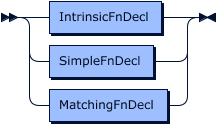Let's look at the types of functions we have.

## Intrinsic Functions​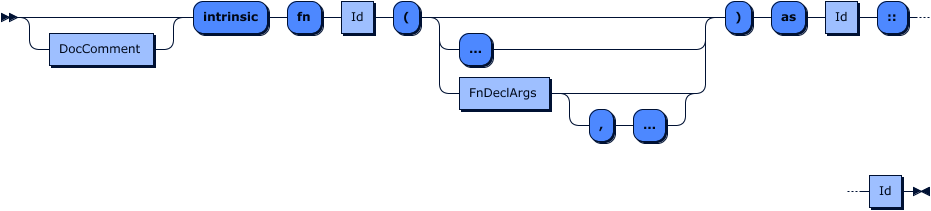Intrinsic functions represent builtin or pre-defined functions implemented in the Rust programming language that are a builtin component of the Tremor project and are provided out of the box.

The function reference in this documentation set, for example, is generated from the documentation provided in the standard library. The standard library is primarily composed of intrinsic or bulitin functions.

## Standard functions​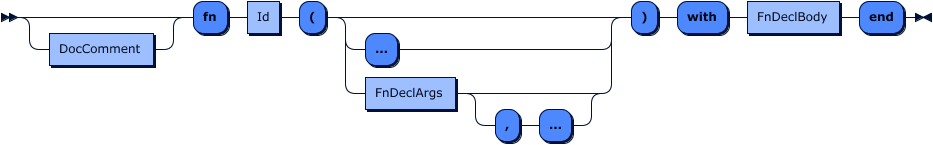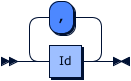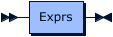Standard functions are functions that take a given number of arguments, each with a name. This function can be tail-recursive. An example would be:

``## This function adds two values togetherfn add(a, b) with  a + bend``

### Variable arguments​

It is possible to use variable arguments by appending a final `...` ellipsis in a standard function definition. The anonymous arguments will be provided via the `args` keyword.

### Recursion​

Tail-recursion is provided for fixed arity ( number of arguments ) standard functions.

Tremor imposes a restriction in recursion depth. As Tremor is an event processing system it is not desirable to have long-running functions that block events from being processed through the system.

``use std::array;fn sum_(e, es) with  let l = array::len(es);  match l of    case l when l > 0 => let a = es, recur(e + es, es[1:l])    default => e  endend;fn sum(...) with  sum_(0, args)end``

## Match functions​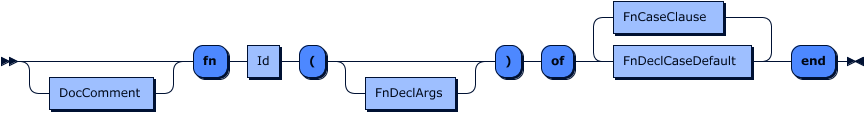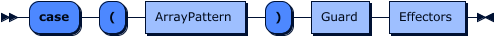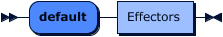Since matching and extracting are a core functionality for tremor matching on function arguments is directly supported.

The same patterns that are used in `match` can be used in function cases including extractors. If any extracting pattern is used and matches the function argument will be replaced by the result of the extraction.

``## calculates the fibonacci sequencefn fib_(a, b, n) of  case (a, b, n) when n > 0 => recur(b, a + b, n - 1)  default => aend;## calculates the fibonacci sequencefn fib(n) with  fib_(0, 1, n)end;``